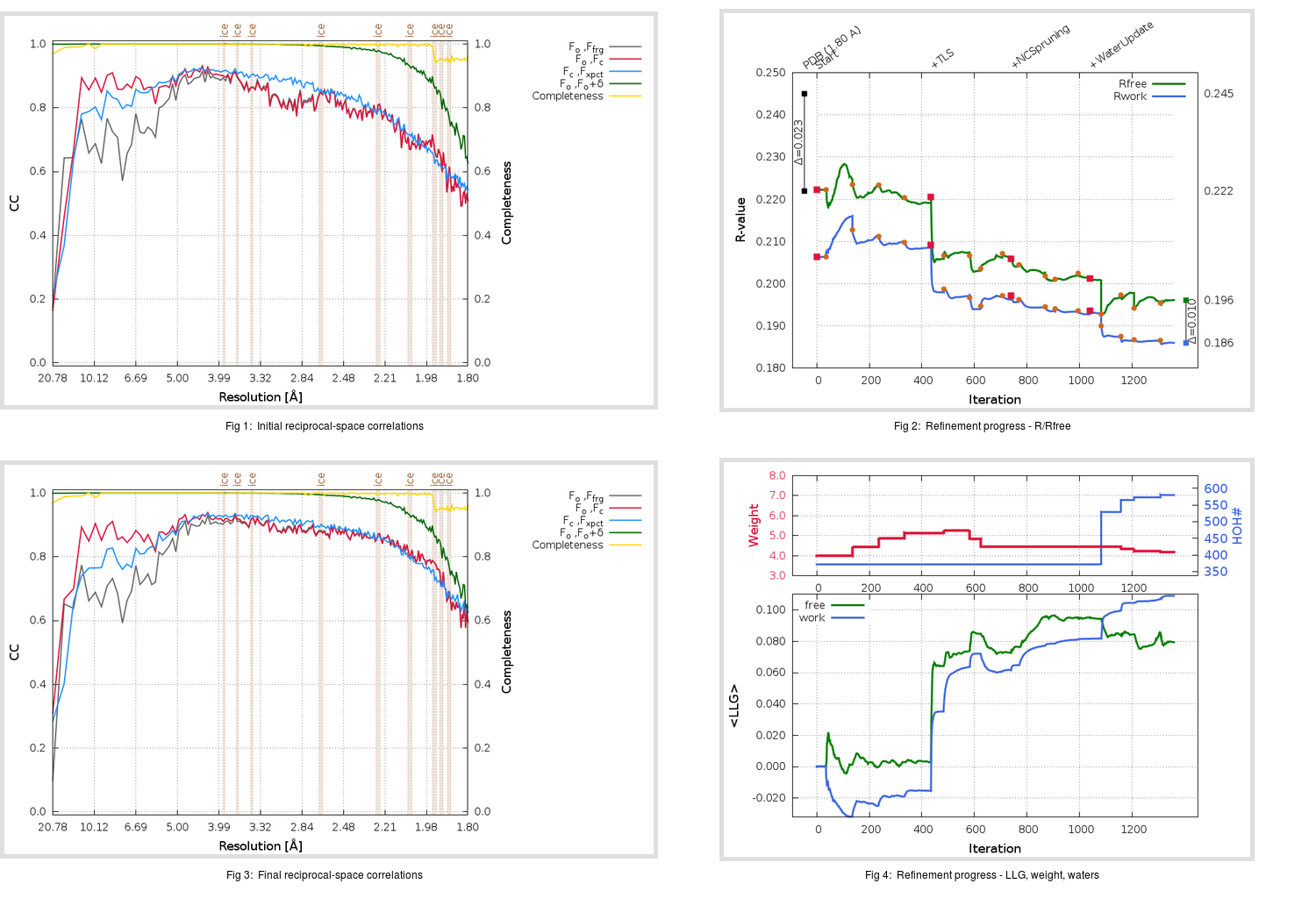Content:

```    Diffraction limits & principal axes of ellipsoid fitted to diffraction cut-off surface:
2.119         0.9015   0.0000  -0.4327       0.700 a* - 0.714 c*
1.823         0.0000   1.0000   0.0000       b*
1.734         0.4327   0.0000   0.9015       0.394 a* + 0.919 c*
```

## Deposited

` `
 Date deposited Date data collection Resolution R, Rfree 20010731 20000731 1.80 0.2220 0.2450

Molprobity (CCP4 7.0 version) summary:

```Ramachandran outliers =   0.67 %
favored =  93.78 %
Rotamer outliers      =   2.02 %
C-beta deviations     =     1
Clashscore            =   9.95
RMS(bonds)            =   0.0066
RMS(angles)           =   1.38
MolProbity score      =   2.16
Resolution            =   1.80
R-work                =   0.2220
R-free                =   0.2450
```

```Number of waters      =   372

<B> (all atoms) =   38.43 ( sd =   12.57 ) for       7838 non-hydrogen atoms
<B>   (protein) =   38.13 ( sd =   12.46 ) for       7391 non-hydrogen atoms
<B>     (water) =   39.94 ( sd =   10.43 ) for        372 non-hydrogen atoms
<B>    (others) =   60.14 ( sd =   13.11 ) for         75 non-hydrogen atoms

B min/max       (all non-hydrogen atoms) =   15.58 /   86.67
B min/max   (protein non-hydrogen atoms) =   15.58 /   86.67
B min/max     (water non-hydrogen atoms) =   19.65 /   68.78
B min/max     (other non-hydrogen atoms) =   27.40 /   73.01
```

## BUSTER (re-)refinement

` `

Molprobity (CCP4 7.0 version) summary:

```Ramachandran outliers =   0.33 %
favored =  96.89 %
Rotamer outliers      =   2.52 %
C-beta deviations     =     2
Clashscore            =   2.79
RMS(bonds)            =   0.0122
RMS(angles)           =   1.59
MolProbity score      =   1.56
Resolution            =   1.80
R-work                =   0.1860
R-free                =   0.1962
```

```Number of waters      =   580

<B> (all atoms) =   43.72 ( sd =   13.88 ) for       8046 non-hydrogen atoms
<B>   (protein) =   43.04 ( sd =   13.73 ) for       7391 non-hydrogen atoms
<B>     (water) =   50.22 ( sd =   13.04 ) for        580 non-hydrogen atoms
<B>    (others) =   60.16 ( sd =   11.45 ) for         75 non-hydrogen atoms

B min/max       (all non-hydrogen atoms) =   19.56 /  120.80
B min/max   (protein non-hydrogen atoms) =   19.56 /  120.80
B min/max     (water non-hydrogen atoms) =   22.35 /  101.50
B min/max     (other non-hydrogen atoms) =   29.76 /   73.48
```

Refinement progression:Results:

` `
 File Remark 1JOU_aB_refine.01_04_refine.pdb.gz exact refinement commands are in header 1JOU_aB_refine.01_04_refine.mtz.gz including original deposited data and several re-refinement map coefficients 1JOU_aB_refine.01_04_BUSTER_model.cif.gz including any non-standard compound restraints 1JOU_aB_refine.01_04_BUSTER_refln.cif.gz